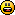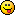<![CDATA[Math Is Fun Forum / Euler's forumula]]> 2006-04-10T01:05:15Z FluxBB http://www.mathisfunforum.com/viewtopic.php?id=3204 <![CDATA[Re: Euler's forumula]]> p²-10p(1-p)+5(1-p)²=0 corrected
16p²-20p+5=0

p= (20±√80)/32 = (5+√5)/8 = (10+2√5)/16

1-p= (3-√5)/8

cos(18°) = √p or=+√p

since no one can solve that out

i just compute  cos(36°) = 2p-1 = (1+√5)/4 , cos²(36°)= 1-previous²
and

]]>
http://www.mathisfunforum.com/profile.php?id=3192 2006-04-10T01:05:15Z http://www.mathisfunforum.com/viewtopic.php?pid=33182#p33182
<![CDATA[Re: Euler's forumula]]> cos(180°)=
cos(45° )[sup]4[/sup]-6cos(45° )[sup]2[/sup]sin(45°)²+sin(45°)[sup]4[/sup]
=1/4-6(1/4)+1/4=-1

0=cos(90°)=cos[sup]5[/sup](18°) -10cos³(18°)sin²(18°)+5cos(18°)sin[sup]4[/sup](18°)

define p=cos²(18°) hence sin²(18°)=1-p

p²-10p(1-p)+(1-p)²=0

]]>
http://www.mathisfunforum.com/profile.php?id=3192 2006-04-09T16:24:17Z http://www.mathisfunforum.com/viewtopic.php?pid=33160#p33160
<![CDATA[Re: Euler's forumula]]> It really has an great application!!
Beyond simple trigonometric formulas

It can be used to derive cos(nθ) and sin(nθ) by cos(θ) and sin(θ).

...

last part ,+ -uncertain,

when n is even,

or

when n is odd]]>
http://www.mathisfunforum.com/profile.php?id=3192 2006-04-08T06:09:44Z http://www.mathisfunforum.com/viewtopic.php?pid=33096#p33096
<![CDATA[Re: Euler's forumula]]> Never mind. we just have different angles]]>
http://www.mathisfunforum.com/profile.php?id=3192 2006-03-24T13:21:01Z http://www.mathisfunforum.com/viewtopic.php?pid=32269#p32269
<![CDATA[Re: Euler's forumula]]> George, I agree.  And actually, I agreed before you ever backed up your statement.  I really just wanted you to start showing what you said instead of just stating it.But I think you missed the entire point of this topic.  There is only one trig formula, and if you accept this formula, then you can generate every single other trig identity.

We aren't proving things.  Now I have used the word prove before, but what I meant was "assuming that e^iθ = cosθ + isinθ, prove that ...."  Sorry if the wording I used was bad, I should have used "show" instead of "prove."

]]>
http://www.mathisfunforum.com/profile.php?id=2143 2006-03-24T04:40:49Z http://www.mathisfunforum.com/viewtopic.php?pid=32216#p32216
<![CDATA[Re: Euler's forumula]]> because in order to prove Euler's formula, we commonly use Taylor series.

And both Sine and Cosine Taylor series are derived via making derivatives (i don't know how to use a single verb to say it) of themselves.

Sine's derivative's proof has an essential part:

Sin(x+Δx) - Sin(x) = 2Sin((x+Δx-x)/2)Cos((x+Δx+x)/2)=2Sin(Δx/2)Cos(x+Δx/2)

More or less does cosine's]]>
http://www.mathisfunforum.com/profile.php?id=3192 2006-03-24T01:31:53Z http://www.mathisfunforum.com/viewtopic.php?pid=32202#p32202
<![CDATA[Re: Euler's forumula]]> And why exactly is it circular, George?

]]>
http://www.mathisfunforum.com/profile.php?id=2143 2006-03-23T21:13:19Z http://www.mathisfunforum.com/viewtopic.php?pid=32190#p32190
<![CDATA[Re: Euler's forumula]]> Though a very good angle to memorize those formulas]]>
http://www.mathisfunforum.com/profile.php?id=3192 2006-03-23T15:22:44Z http://www.mathisfunforum.com/viewtopic.php?pid=32172#p32172
<![CDATA[Re: Euler's forumula]]> Circular proof, i am sorry to say

Why don't you explore other applications, to which cannot be solved simply within trigonometric formulas?

eg.

Sin[A] Sin[b] Sin[C]

]]>
http://www.mathisfunforum.com/profile.php?id=3192 2006-03-23T15:21:31Z http://www.mathisfunforum.com/viewtopic.php?pid=32171#p32171
<![CDATA[Re: Euler's forumula]]> Actually, let's skip cos(x) = cos(-x) and sin(x) = -sin(-x) for now.  I believe they will come up soon enough.

Prove that cos(a+b) = cos(a)cos(b) - sin(a)sin(b).

]]>
http://www.mathisfunforum.com/profile.php?id=2143 2006-03-22T01:22:10Z http://www.mathisfunforum.com/viewtopic.php?pid=32052#p32052
<![CDATA[Re: Euler's forumula]]> One property used in that proof is cos(x) = cos(-x) and sin(x) = -sin(x).  I have yet to find a way to prove these using e^iθ, but for the moment, do so using the definitions of sine and cosine.

]]>
http://www.mathisfunforum.com/profile.php?id=2143 2006-03-20T04:32:43Z http://www.mathisfunforum.com/viewtopic.php?pid=31849#p31849
<![CDATA[Re: Euler's forumula]]> Absolutely right, George Y.
.
By Abraham de Moivre's theorem,

(Cosx+iSinx)(Cosx-iSinx)=Cos²x - (i²)Sin²x = Cos²x - (-1)Sin²x
= Cos²x + Sin²x

Therefore, Cos²x + Sin²x=1.

]]>
http://www.mathisfunforum.com/profile.php?id=682 2006-03-20T03:57:25Z http://www.mathisfunforum.com/viewtopic.php?pid=31847#p31847
<![CDATA[Re: Euler's forumula]]> e^ix e^-ix = (cosx+isinx)(cosx-isinx) < sin(-x) =-sinx > = (cos^2[x] - i^2 sin^2[x])=(cos[x])^2+(sin[x])^2

where e^ix e^-ix =1

]]>
http://www.mathisfunforum.com/profile.php?id=3192 2006-03-20T02:56:48Z http://www.mathisfunforum.com/viewtopic.php?pid=31842#p31842
<![CDATA[Re: Euler's forumula]]> Nicely done.  Now I suggest we go after cos²θ + sin²θ = 1, as that will most likely be a useful property to use for other identites later on.

If no one gets it by tomorrow, I'll post a hint.

]]>
http://www.mathisfunforum.com/profile.php?id=2143 2006-03-19T17:08:16Z http://www.mathisfunforum.com/viewtopic.php?pid=31825#p31825
<![CDATA[Re: Euler's forumula]]> Yes, I noticed that. OK, I'll shamelessly steal your LaTeX and alter it for my own purposes then.]]>
http://www.mathisfunforum.com/profile.php?id=641 2006-03-19T16:51:01Z http://www.mathisfunforum.com/viewtopic.php?pid=31824#p31824